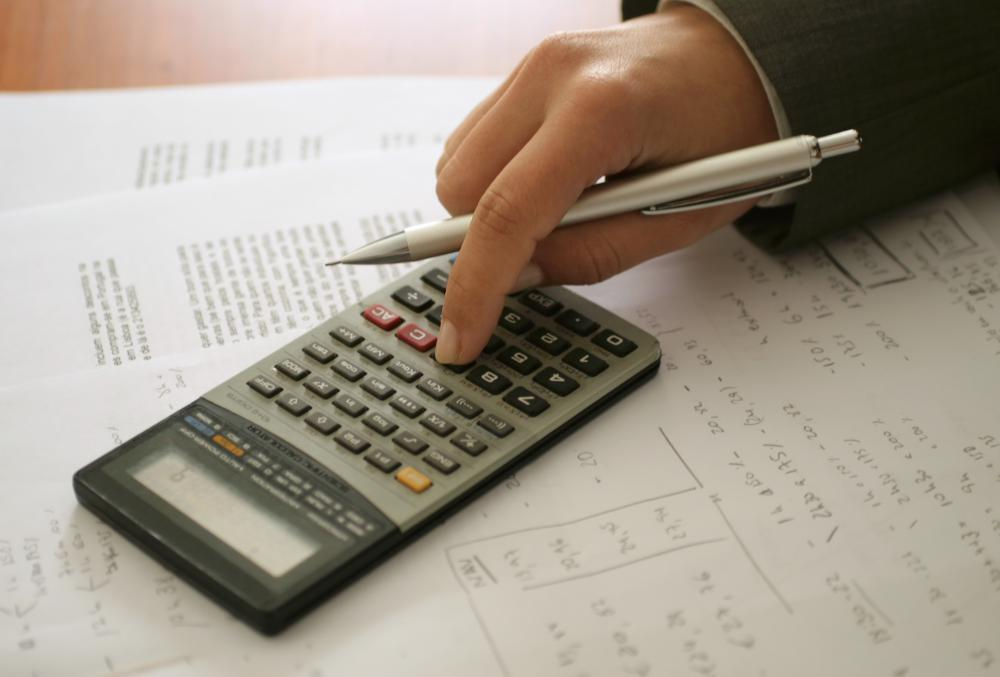# What is Price Variance?

Victoria Blackburn

Price variance is a financial concept that is used in management accounting. Management accounting, or budgeting as it is commonly referred to, is the application of accounting methods to real business issues. Budgeting turns financial information into a form that is more readily useful for making everyday business decisions. Variance theory and more specifically, price variance, calculates the difference between a budgeted and a planned amount, enabling managers to come up with their estimated and actual profits.To calculate the price variance of any factor, estimated price is subtracted from the actual price and this number is multiplied by the actual quantity.

To calculate the price variance of any factor, whether it is labor, materials or sales price, the approach is the same. The estimated price is subtracted from the actual price and this number is multiplied by the actual quantity. This shows the difference between what was expected and what was actually paid. Next, this figure, which represents the variance or change, is multiplied by the amount actually used, to give meaning to the specific project.

There are many different uses for implementing the price variance formula. First, it can be used by a manager to find out the difference between what was budgeted to be spent on materials and what was actually spent. By calculating the difference in cost and then multiplying by the actual amount used, the manager can get a full picture of how much money was spent and therefore be able to report if the project was over or under budget. The same can be said for calculating the price variance of labor or the change in the selling price of a product.

Price variance is fundamental to the analysis of budgets. Without using the price variance method to track the difference between planned spending and actual spending, the preparation of a budget becomes a useless pursuit. This is because this method acts as a way to check performance. For instance, a negative price variance means that the actual costs were less than expected and this is favorable. A positive price variance means that actual costs were more than budgeted or expected; this is a negative outcome as more is spent than was planned.

Price variance indicates when more needs to be done to reign in spending. This concept is used in various industries as a complement to budgeting models. It is also a good way to track the performance of key personnel if an important part of their function is to keep price levels within set limits.

## You might also Like